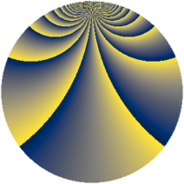# Properties

 Label 1805.2.baLevel $1805$ Weight $2$ Character orbit 1805.ba Rep. character $\chi_{1805}(49,\cdot)$ Character field $\Q(\zeta_{114})$ Dimension $6768$ Sturm bound $380$

# Related objects

## Defining parameters

 Level: $$N$$ $$=$$ $$1805 = 5 \cdot 19^{2}$$ Weight: $$k$$ $$=$$ $$2$$ Character orbit: $$[\chi]$$ $$=$$ 1805.ba (of order $$114$$ and degree $$36$$) Character conductor: $$\operatorname{cond}(\chi)$$ $$=$$ $$1805$$ Character field: $$\Q(\zeta_{114})$$ Sturm bound: $$380$$

## Dimensions

The following table gives the dimensions of various subspaces of $$M_{2}(1805, [\chi])$$.

Total New Old
Modular forms 6912 6912 0
Cusp forms 6768 6768 0
Eisenstein series 144 144 0

## Trace form

 $$6768 q - 262 q^{4} - 37 q^{5} - 64 q^{6} - 258 q^{9} + O(q^{10})$$ $$6768 q - 262 q^{4} - 37 q^{5} - 64 q^{6} - 258 q^{9} - 93 q^{10} - 72 q^{11} - 120 q^{14} - 34 q^{15} + 110 q^{16} - 78 q^{19} + 2 q^{20} - 56 q^{21} - 86 q^{24} - 37 q^{25} - 32 q^{26} - 6 q^{29} - 164 q^{30} - 108 q^{31} - 78 q^{34} - 57 q^{35} + 86 q^{36} + 72 q^{39} - 48 q^{40} - 60 q^{41} - 92 q^{44} + 19 q^{45} - 94 q^{46} + 304 q^{49} + 78 q^{50} + 40 q^{51} - 42 q^{54} - 27 q^{55} - 130 q^{56} - 114 q^{59} - 42 q^{60} - 94 q^{61} + 228 q^{64} + 62 q^{65} - 62 q^{66} - 876 q^{69} + 204 q^{70} - 76 q^{71} - 138 q^{74} - 210 q^{75} - 572 q^{76} - 102 q^{79} - 188 q^{80} + 136 q^{81} - 94 q^{84} - 43 q^{85} - 768 q^{86} - 204 q^{89} - 6 q^{90} - 160 q^{91} - 196 q^{94} - 290 q^{95} + 242 q^{96} - 20 q^{99} + O(q^{100})$$

## Decomposition of $$S_{2}^{\mathrm{new}}(1805, [\chi])$$ into newform subspaces

The newforms in this space have not yet been added to the LMFDB.